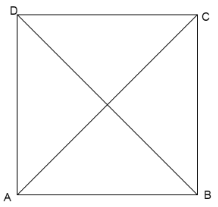Prove that the points A(1,7), B(4,2), C(-1,-1), D(-4,4) are the vertices of a square.Verified
149.4k+ views
Hint: A square is a four sided regular shape having four equal sides and four equal angles (${90^0}$) with two diagonal length = $\sqrt 2 a$ where a is the length of the side.To find the distance between two coordinates (a,b) and (c,d), we need to calculate using Euclidean distance formula which is $\sqrt {{{(c - a)}^2} + {{(d - b)}^2}}$

Here they have provided all the four vertices as coordinates. We need to check the property whether they are lying in the same distance using the above formula. And check both the diagonal lengths are equal and = $\sqrt 2$ times the side length.
Step by step solution:
Step 1: Finding distance between first two points A(1,7) and B(4,2).
a=1 , b=7 , c=4 , d=2
Distance
$= \sqrt {{{(c - a)}^2} + {{(d - b)}^2}} \\ = \sqrt {{{(4 - 1)}^2} + {{(2 - 7)}^2}} \\ = \sqrt {{3^2} + {{( - 5)}^2}} \\ = \sqrt {9 + 25} \\ = \sqrt {34} \\$
Now we need to find all the other distances and check whether they are all equal to $\sqrt {34}$ .
Step 2: Finding distance between B(4,2) and C(-1,-1)
a=4 , b=2 , c=-1 , d=-1
Distance = $\sqrt {{{(c - a)}^2} + {{(d - b)}^2}}$
$= \sqrt {{{( - 1 - 4)}^2} + {{( - 1 - 2)}^2}} \\ = \sqrt {{{( - 5)}^2} + {{( - 3)}^2}} \\ = \sqrt {25 + 9} \\ = \sqrt {34} \\$
We have found this distance is the same as before.
Step 3: Finding distance between C(-1,-1) and D(-4,4)
a=-1 , b=-1 , c=-4 , d=4
Distance = $\sqrt {{{(c - a)}^2} + {{(d - b)}^2}}$
$= \sqrt {{{( - 4 - ( - 1))}^2} + {{(4 - ( - 1))}^2}} \\ = \sqrt {{{( - 3)}^2} + {{(5)}^2}} \\ = \sqrt {9 + 25} \\ = \sqrt {34} \\$
Which is again the same as above.
Step 3: Now remaining is o find distance between D(-4,4) and A(1,7)
A=-4 , b=4 , c=1 , d=7
Distance = $\sqrt {{{(c - a)}^2} + {{(d - b)}^2}}$
$= \sqrt {{{(1 - ( - 4))}^2} + {{(7 - 4)}^2}} \\ = \sqrt {{{(5)}^2} + {{(3)}^2}} \\ = \sqrt {25 + 9} \\ = \sqrt {34} \\$
Thus we have found the lengths of all the four sides which are all the same.
Step 4: Now to check the diagonals AC and BD are $\sqrt 2$ times the side length=6.
Distance between A(1,7) and C(-1,-1) = $\sqrt {{{(c - a)}^2} + {{(d - b)}^2}}$
a=1 , b=7 , c=-1 , d=1
$= \sqrt {{{( - 1 - 1)}^2} + {{( - 1 - 7)}^2}} \\ = \sqrt {{{( - 2)}^2} + {{( - 8)}^2}} \\ = \sqrt {4 + 64} \\ = \sqrt {2(2 + 32)} \\ = \sqrt 2 \times \sqrt {34} \\$
Which is $\sqrt 2$ times the side length.
Step 5: Now the other diagonal BD
Distance between B(4,2) and D(-4,4)= $\sqrt {{{(c - a)}^2} + {{(d - b)}^2}}$
a=4 , b=2 , c=-4 , d=4
$= \sqrt {{{( - 4 - 4)}^2} + {{(4 - 2)}^2}} \\ = \sqrt {{{( - 8)}^2} + {{(2)}^2}} \\ = \sqrt {64 + 4} \\ = \sqrt {2(32 + 2)} \\ = \sqrt 2 \times \sqrt {34} \\$
Which is$\sqrt 2$ times the length of the side.
Hence proved.
Thus all four sides are of equal length and two diagonals are equal and $\sqrt 2$ times the sides length. Thus given points are vertices of a square.
Given points are vertices of a square.

Note: Students are likely to get confused while calculating the value inside the square root, it is recommended always to substitute the value in the formula and then solve, also to check the answer of lengths, always check the value should not be negative because they are lengths of sides and length can never be negative.